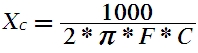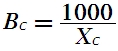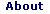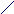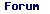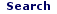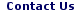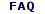Mon - 01 Jun 2020

WELCOME TO RFCABLES.ORG

## Inductive Reactance and Admittance Calculator

 Enter F and L values to Calculate the XL and BL. F (GHz) L (nH) XL (ohms) BL (m-mhos)
 Enter XL and F values to Calculate the L and BL. XL(ohms) F (GHz) L (nH) BL (m-mhos)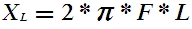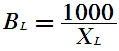## Capacitive Reactance and Admittance Calculator

 Enter F and C values to Calculate the XC and BC. F (GHz) C (pF) XC (ohms) BC (m-mhos)
 Enter XC and F values to Calculate the C and BC. XC(ohms) F (GHz) C (pF) BC (m-mhos)